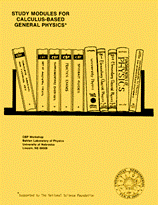Instructional Materials in Physics and AstronomyCalculus-Based General Physics

Planar Motion

Date of this Version

1975

From Study Modules for Calculus-Based General Physics
Reproduction rights granted.

Abstract

Enough of this physics where things move along straight lines only! We know that most interesting real-life motions involve curves of many and varied shapes. This module extends your understanding of kinematics from one dimension to two dimensions. To accomplish this, you will combine your knowledge of calculus and vectors with concepts like position, displacement, velocity, speed, and acceleration.

Two important applications that will be utilized many times in later modules are covered here. First is the motion of a particle experiencing constant acceleration, e.g., a baseball in flight. Second is the motion of a particle in a circular path with a constant speed, e.g., an earth satellite in circular orbit.

COinS